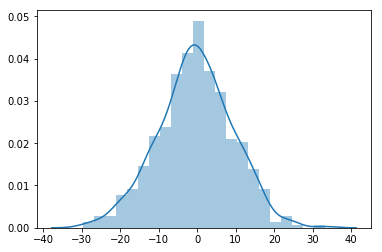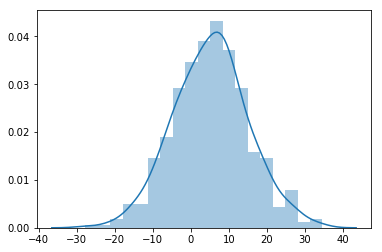# T-Test

TTest is a statical hypothesis test, it is used for quantitative analysis. It is of two types,When we perform a t-test, we usually trying to find evidence of a significant difference between population means (2-sample t) or between the population mean and a hypothesized value (1-sample t). Thus in other words,

1 Sample: It will compare the sample mean with population mean.

2 Sample: It compare the Independent sample or dependent sample.

from scipy import stats
rvs1 = stats.norm.rvs(loc=0,scale=10,size=500)
sns.distplot(rvs1)rvs2 = stats.norm.rvs(loc=5,scale=10,size=500)
sns.distplot(rvs2)stats.ttest_ind(rvs1,rvs2)
`Ttest_indResult(statistic=-9.2606939302039226, pvalue=1.2094782231229125e-19)`
Following are the assumptions of T test:
1-The assumption for a t-test is that the scale of measurement applied to the data collected follows a continuous or ordinal scale.
2-The second assumption made is that of a simple random sample, that the data is collected from a representative, randomly selected portion of the total population.
3-The third assumption is that the data, when plotted, results in a normal distribution, bell-shaped distribution curve.
4-The fourth assumption is that a reasonably large sample size is used. A larger sample size means that the distribution of results should approach a normal bell-shaped curve.

5-The final assumption is homogeneity of variance. Homogeneous, or equal, variance exists when the standard deviations of samples are approximately equal.

Note: The assumption of homogeneity of variance is that the variance within each of the populations is equal.